# 6.4 Graphs of logarithmic functions  (Page 8/8)

 Page 8 / 8

## Verbal

The inverse of every logarithmic function is an exponential function and vice-versa. What does this tell us about the relationship between the coordinates of the points on the graphs of each?

Since the functions are inverses, their graphs are mirror images about the line $\text{\hspace{0.17em}}y=x.\text{\hspace{0.17em}}$ So for every point $\text{\hspace{0.17em}}\left(a,b\right)\text{\hspace{0.17em}}$ on the graph of a logarithmic function, there is a corresponding point $\text{\hspace{0.17em}}\left(b,a\right)\text{\hspace{0.17em}}$ on the graph of its inverse exponential function.

What type(s) of translation(s), if any, affect the range of a logarithmic function?

What type(s) of translation(s), if any, affect the domain of a logarithmic function?

Shifting the function right or left and reflecting the function about the y-axis will affect its domain.

Consider the general logarithmic function $\text{\hspace{0.17em}}f\left(x\right)={\mathrm{log}}_{b}\left(x\right).\text{\hspace{0.17em}}$ Why can’t $\text{\hspace{0.17em}}x\text{\hspace{0.17em}}$ be zero?

Does the graph of a general logarithmic function have a horizontal asymptote? Explain.

No. A horizontal asymptote would suggest a limit on the range, and the range of any logarithmic function in general form is all real numbers.

## Algebraic

For the following exercises, state the domain and range of the function.

$f\left(x\right)={\mathrm{log}}_{3}\left(x+4\right)$

$h\left(x\right)=\mathrm{ln}\left(\frac{1}{2}-x\right)$

Domain: $\text{\hspace{0.17em}}\left(-\infty ,\frac{1}{2}\right);\text{\hspace{0.17em}}$ Range: $\text{\hspace{0.17em}}\left(-\infty ,\infty \right)$

$g\left(x\right)={\mathrm{log}}_{5}\left(2x+9\right)-2$

$h\left(x\right)=\mathrm{ln}\left(4x+17\right)-5$

Domain: $\text{\hspace{0.17em}}\left(-\frac{17}{4},\infty \right);\text{\hspace{0.17em}}$ Range: $\text{\hspace{0.17em}}\left(-\infty ,\infty \right)$

$f\left(x\right)={\mathrm{log}}_{2}\left(12-3x\right)-3$

For the following exercises, state the domain and the vertical asymptote of the function.

$\text{\hspace{0.17em}}f\left(x\right)={\mathrm{log}}_{b}\left(x-5\right)$

Domain: $\text{\hspace{0.17em}}\left(5,\infty \right);\text{\hspace{0.17em}}$ Vertical asymptote: $\text{\hspace{0.17em}}x=5$

$\text{\hspace{0.17em}}g\left(x\right)=\mathrm{ln}\left(3-x\right)$

$\text{\hspace{0.17em}}f\left(x\right)=\mathrm{log}\left(3x+1\right)$

Domain: $\text{\hspace{0.17em}}\left(-\frac{1}{3},\infty \right);\text{\hspace{0.17em}}$ Vertical asymptote: $\text{\hspace{0.17em}}x=-\frac{1}{3}$

$\text{\hspace{0.17em}}f\left(x\right)=3\mathrm{log}\left(-x\right)+2$

$\text{\hspace{0.17em}}g\left(x\right)=-\mathrm{ln}\left(3x+9\right)-7$

Domain: $\text{\hspace{0.17em}}\left(-3,\infty \right);\text{\hspace{0.17em}}$ Vertical asymptote: $\text{\hspace{0.17em}}x=-3$

For the following exercises, state the domain, vertical asymptote, and end behavior of the function.

$f\left(x\right)=\mathrm{ln}\left(2-x\right)$

$f\left(x\right)=\mathrm{log}\left(x-\frac{3}{7}\right)$

Domain: $\left(\frac{3}{7},\infty \right)$ ;
Vertical asymptote: $x=\frac{3}{7}$ ; End behavior: as $x\to {\left(\frac{3}{7}\right)}^{+},f\left(x\right)\to -\infty$ and as $x\to \infty ,f\left(x\right)\to \infty$

$h\left(x\right)=-\mathrm{log}\left(3x-4\right)+3$

$g\left(x\right)=\mathrm{ln}\left(2x+6\right)-5$

Domain: $\left(-3,\infty \right)$ ; Vertical asymptote: $x=-3$ ;
End behavior: as $x\to -{3}^{+}$ , $f\left(x\right)\to -\infty$ and as $x\to \infty$ , $f\left(x\right)\to \infty$

$f\left(x\right)={\mathrm{log}}_{3}\left(15-5x\right)+6$

For the following exercises, state the domain, range, and x - and y -intercepts, if they exist. If they do not exist, write DNE.

$h\left(x\right)={\mathrm{log}}_{4}\left(x-1\right)+1$

Domain: $\text{\hspace{0.17em}}\left(1,\infty \right);\text{\hspace{0.17em}}$ Range: $\text{\hspace{0.17em}}\left(-\infty ,\infty \right);\text{\hspace{0.17em}}$ Vertical asymptote: $\text{\hspace{0.17em}}x=1;\text{\hspace{0.17em}}$ x -intercept: $\text{\hspace{0.17em}}\left(\frac{5}{4},0\right);\text{\hspace{0.17em}}$ y -intercept: DNE

$f\left(x\right)=\mathrm{log}\left(5x+10\right)+3$

$g\left(x\right)=\mathrm{ln}\left(-x\right)-2$

Domain: $\text{\hspace{0.17em}}\left(-\infty ,0\right);\text{\hspace{0.17em}}$ Range: $\text{\hspace{0.17em}}\left(-\infty ,\infty \right);\text{\hspace{0.17em}}$ Vertical asymptote: $\text{\hspace{0.17em}}x=0;\text{\hspace{0.17em}}$ x -intercept: $\text{\hspace{0.17em}}\left(-{e}^{2},0\right);\text{\hspace{0.17em}}$ y -intercept: DNE

$f\left(x\right)={\mathrm{log}}_{2}\left(x+2\right)-5$

$h\left(x\right)=3\mathrm{ln}\left(x\right)-9$

Domain: $\text{\hspace{0.17em}}\left(0,\infty \right);\text{\hspace{0.17em}}$ Range: $\text{\hspace{0.17em}}\left(-\infty ,\infty \right);\text{\hspace{0.17em}}$ Vertical asymptote: $\text{\hspace{0.17em}}x=0;\text{\hspace{0.17em}}$ x -intercept: $\text{\hspace{0.17em}}\left({e}^{3},0\right);\text{\hspace{0.17em}}$ y -intercept: DNE

## Graphical

For the following exercises, match each function in [link] with the letter corresponding to its graph.

$d\left(x\right)=\mathrm{log}\left(x\right)$

$f\left(x\right)=\mathrm{ln}\left(x\right)$

B

$g\left(x\right)={\mathrm{log}}_{2}\left(x\right)$

$h\left(x\right)={\mathrm{log}}_{5}\left(x\right)$

C

$j\left(x\right)={\mathrm{log}}_{25}\left(x\right)$

For the following exercises, match each function in [link] with the letter corresponding to its graph.

$f\left(x\right)={\mathrm{log}}_{\frac{1}{3}}\left(x\right)$

B

$g\left(x\right)={\mathrm{log}}_{2}\left(x\right)$

$h\left(x\right)={\mathrm{log}}_{\frac{3}{4}}\left(x\right)$

C

For the following exercises, sketch the graphs of each pair of functions on the same axis.

$f\left(x\right)=\mathrm{log}\left(x\right)\text{\hspace{0.17em}}$ and $\text{\hspace{0.17em}}g\left(x\right)={10}^{x}$

$f\left(x\right)=\mathrm{log}\left(x\right)\text{\hspace{0.17em}}$ and $\text{\hspace{0.17em}}g\left(x\right)={\mathrm{log}}_{\frac{1}{2}}\left(x\right)$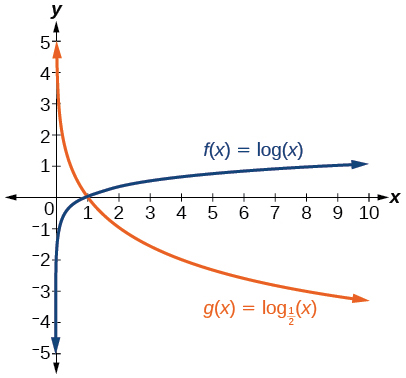$f\left(x\right)={\mathrm{log}}_{4}\left(x\right)\text{\hspace{0.17em}}$ and $\text{\hspace{0.17em}}g\left(x\right)=\mathrm{ln}\left(x\right)$

$f\left(x\right)={e}^{x}\text{\hspace{0.17em}}$ and $\text{\hspace{0.17em}}g\left(x\right)=\mathrm{ln}\left(x\right)$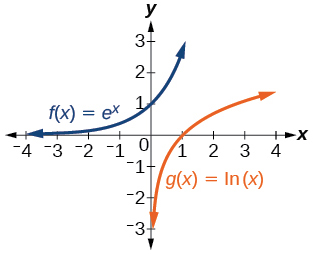For the following exercises, match each function in [link] with the letter corresponding to its graph.

$f\left(x\right)={\mathrm{log}}_{4}\left(-x+2\right)$

$g\left(x\right)=-{\mathrm{log}}_{4}\left(x+2\right)$

C

$h\left(x\right)={\mathrm{log}}_{4}\left(x+2\right)$

For the following exercises, sketch the graph of the indicated function.

$\text{\hspace{0.17em}}f\left(x\right)={\mathrm{log}}_{2}\left(x+2\right)$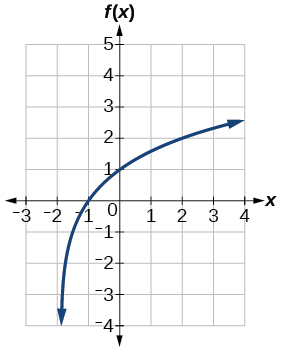$\text{\hspace{0.17em}}f\left(x\right)=2\mathrm{log}\left(x\right)$

$\text{\hspace{0.17em}}f\left(x\right)=\mathrm{ln}\left(-x\right)$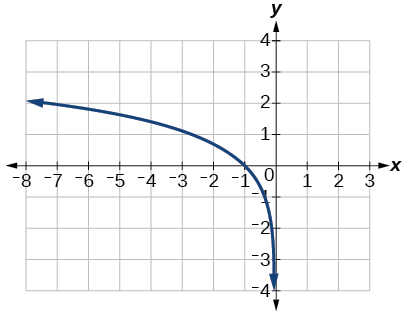$g\left(x\right)=\mathrm{log}\left(4x+16\right)+4$

$g\left(x\right)=\mathrm{log}\left(6-3x\right)+1$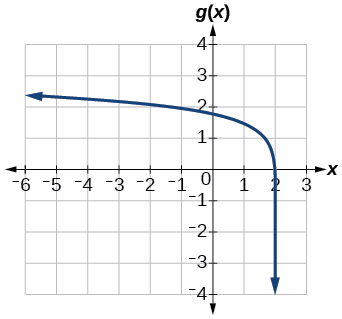$h\left(x\right)=-\frac{1}{2}\mathrm{ln}\left(x+1\right)-3$

For the following exercises, write a logarithmic equation corresponding to the graph shown.

Use $\text{\hspace{0.17em}}y={\mathrm{log}}_{2}\left(x\right)\text{\hspace{0.17em}}$ as the parent function.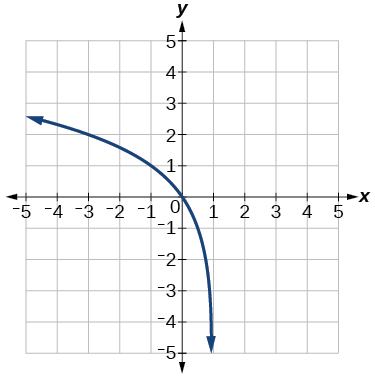$\text{\hspace{0.17em}}f\left(x\right)={\mathrm{log}}_{2}\left(-\left(x-1\right)\right)$

Use $\text{\hspace{0.17em}}f\left(x\right)={\mathrm{log}}_{3}\left(x\right)\text{\hspace{0.17em}}$ as the parent function.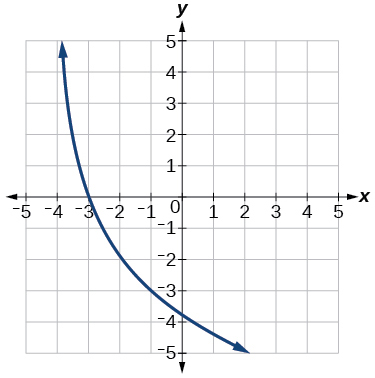Use $\text{\hspace{0.17em}}f\left(x\right)={\mathrm{log}}_{4}\left(x\right)\text{\hspace{0.17em}}$ as the parent function.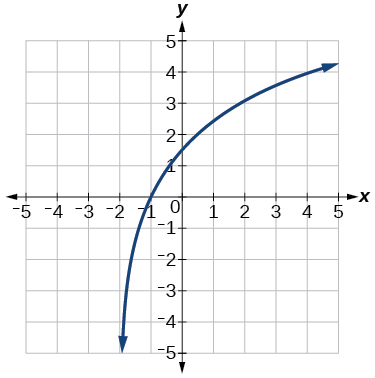$f\left(x\right)=3{\mathrm{log}}_{4}\left(x+2\right)$

Use $\text{\hspace{0.17em}}f\left(x\right)={\mathrm{log}}_{5}\left(x\right)\text{\hspace{0.17em}}$ as the parent function.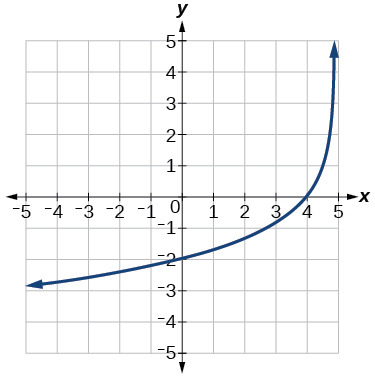## Technology

For the following exercises, use a graphing calculator to find approximate solutions to each equation.

$\mathrm{log}\left(x-1\right)+2=\mathrm{ln}\left(x-1\right)+2$

$x=2$

$\mathrm{log}\left(2x-3\right)+2=-\mathrm{log}\left(2x-3\right)+5$

$\mathrm{ln}\left(x-2\right)=-\mathrm{ln}\left(x+1\right)$

$x\approx \text{2}\text{.303}$

$2\mathrm{ln}\left(5x+1\right)=\frac{1}{2}\mathrm{ln}\left(-5x\right)+1$

$\frac{1}{3}\mathrm{log}\left(1-x\right)=\mathrm{log}\left(x+1\right)+\frac{1}{3}$

$x\approx -0.472$

## Extensions

Let $\text{\hspace{0.17em}}b\text{\hspace{0.17em}}$ be any positive real number such that $\text{\hspace{0.17em}}b\ne 1.\text{\hspace{0.17em}}$ What must $\text{\hspace{0.17em}}{\mathrm{log}}_{b}1\text{\hspace{0.17em}}$ be equal to? Verify the result.

Explore and discuss the graphs of $\text{\hspace{0.17em}}f\left(x\right)={\mathrm{log}}_{\frac{1}{2}}\left(x\right)\text{\hspace{0.17em}}$ and $\text{\hspace{0.17em}}g\left(x\right)=-{\mathrm{log}}_{2}\left(x\right).\text{\hspace{0.17em}}$ Make a conjecture based on the result.

The graphs of $\text{\hspace{0.17em}}f\left(x\right)={\mathrm{log}}_{\frac{1}{2}}\left(x\right)\text{\hspace{0.17em}}$ and $\text{\hspace{0.17em}}g\left(x\right)=-{\mathrm{log}}_{2}\left(x\right)\text{\hspace{0.17em}}$ appear to be the same; Conjecture: for any positive base $\text{\hspace{0.17em}}b\ne 1,$ $\text{\hspace{0.17em}}{\mathrm{log}}_{b}\left(x\right)=-{\mathrm{log}}_{\frac{1}{b}}\left(x\right).$

Prove the conjecture made in the previous exercise.

What is the domain of the function $\text{\hspace{0.17em}}f\left(x\right)=\mathrm{ln}\left(\frac{x+2}{x-4}\right)?\text{\hspace{0.17em}}$ Discuss the result.

Recall that the argument of a logarithmic function must be positive, so we determine where $\text{\hspace{0.17em}}\frac{x+2}{x-4}>0\text{\hspace{0.17em}}$ . From the graph of the function $\text{\hspace{0.17em}}f\left(x\right)=\frac{x+2}{x-4},$ note that the graph lies above the x -axis on the interval $\text{\hspace{0.17em}}\left(-\infty ,-2\right)\text{\hspace{0.17em}}$ and again to the right of the vertical asymptote, that is $\text{\hspace{0.17em}}\left(4,\infty \right).\text{\hspace{0.17em}}$ Therefore, the domain is $\text{\hspace{0.17em}}\left(-\infty ,-2\right)\cup \left(4,\infty \right).$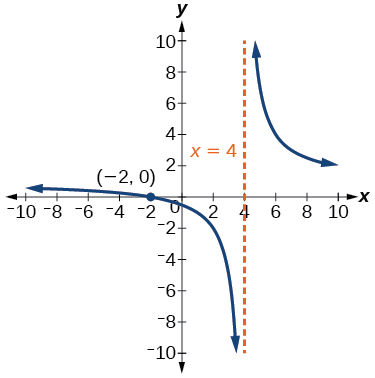Use properties of exponents to find the x -intercepts of the function $\text{\hspace{0.17em}}f\left(x\right)=\mathrm{log}\left({x}^{2}+4x+4\right)\text{\hspace{0.17em}}$ algebraically. Show the steps for solving, and then verify the result by graphing the function.

what are odd numbers
numbers that leave a remainder when divided by 2
Thorben
1,3,5,7,... 99,...867
Thorben
7%2=1, 679%2=1, 866245%2=1
Thorben
the third and the seventh terms of a G.P are 81 and 16, find the first and fifth terms.
if a=3, b =4 and c=5 find the six trigonometric value sin
pls how do I factorize x⁴+x³-7x²-x+6=0
in a function the input value is called
how do I test for values on the number line
if a=4 b=4 then a+b=
a+b+2ab
Kin
commulative principle
a+b= 4+4=8
Mimi
If a=4 and b=4 then we add the value of a and b i.e a+b=4+4=8.
Tariq
what are examples of natural number
an equation for the line that goes through the point (-1,12) and has a slope of 2,3
3y=-9x+25
Ishaq
show that the set of natural numberdoes not from agroup with addition or multiplication butit forms aseni group with respect toaaddition as well as multiplication
x^20+x^15+x^10+x^5/x^2+1
evaluate each algebraic expression. 2x+×_2 if ×=5
if the ratio of the root of ax+bx+c =0, show that (m+1)^2 ac =b^2m
By the definition, is such that 0!=1.why?
(1+cosA+IsinA)(1+cosB+isinB)/(cos@+isin@)(cos$+isin$)
hatdog
Mark
jaks
Ryan

#### Get Jobilize Job Search Mobile App in your pocket Now!ByByBy OpenStaxBy OpenStaxBy OpenStaxBy OpenStaxBy Jessica CollettBy Richley CrapoBy OpenStaxBy Rohini AjayByBy OpenStax